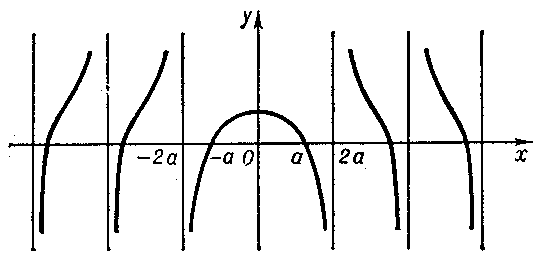##### Actions

A transcendental plane curve which is given in orthogonal Cartesian coordinates by:

$$y=x\operatorname{cotan}\frac{\pi x}{2a},$$

and in polar coordinates by

$$\rho=\frac{a(\pi-2\phi)}{\pi\cos\phi}.$$

A Dinostratus quadratrix has an infinite number of branches (see Fig.), intersecting the $x$-axis at the points $x=\pm a,\pm3a,\pm5a,\ldots,$ with asymptotes $x=\pm2a,\pm4a,\pm6a,\ldots$. The points of intersection with the straight line $y=2a/\pi$ are points of inflection.Figure: d032560a

The first considerations of the quadratrix are attributed to Hippias of Elis (420 B.C.). Dinostratus (second half of the 4th century B.C.) showed that the problem of the quadrature of the circle can be graphically solved with the aid of the quadratrix.